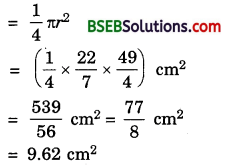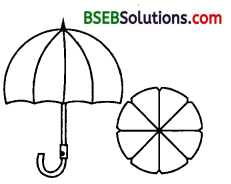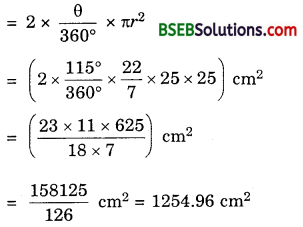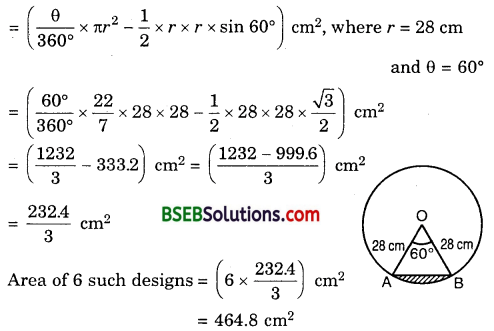# Bihar Board Class 10th Maths Solutions Chapter 12 Areas Related to Circles Ex 12.2

Bihar Board Class 10th Maths Solutions Chapter 12 Areas Related to Circles Ex 12.2 Textbook Questions and Answers.

## BSEB Bihar Board Class 10th Maths Solutions Chapter 12 Areas Related to Circles Ex 12.2Question 1.
Find the area of a sector of a circle with radius 6 cm, if angle of the sector is 60°.
Solution:
We know that the area A of a sector of angle θ in a circle of radius r is given by A = $$\frac{\theta}{360^{\circ}}$$ x πr².
Here, r = 6 cm, θ = 60°
∴ A = $$\left[\frac{60^{\circ}}{360^{\circ}} \times \frac{22}{7} \times 36\right]$$ cm² = $$\left(\frac{132}{7}\right)$$ cm²
= 18.86 cm²

Question 2.
Find the area of a quadrant of a circle whose circumference is 22 cm.
Solution:
Let r cm be the radius of the circle. Then,
Circumference = 22 cm means 2πr = 22
So, 2 x $$\frac { 22 }{ 7 }$$ x r = 22
or r = $$\frac { 7 }{ 2 }$$
So, radius of the circle is $$\frac { 7 }{ 2 }$$ cm.
Area of the quadrant of a circleQuestion 3.
The length of the minute hand of a clock is 14 cm. Find the area swept by the minute hand in 5 minutes.
Solution:
Clearly, minute hand of a clock describes a circle of radius equal to its length, i.e., r = 14 cm.
Since the minute hand rotates through 6° in one minute, therefore area swept by the minute hand in one minute is the area of a sector of angle 6° in a circle of radius 14 cm.
Hence, the required area i.e., the area swept in 5 minutesQuestion 4.
A chord of a circle of radius 10 cm subtends a right angle at the centre. Find the area of the corresponding : (i) minor segment (ii) major sector (Use n = 3.14)
Solution:
Here, r = 10 cm and θ = 90°.
(i) Area of the minor segmentAliter. Area of the minor segment = Area of the sector – Area of right triangle(ii) Area of the major sectorQuestion 5.
In a circle of a radius 21 cm, an arc subtends an angle of 60° at the centre. Find :
(i) the length of the arc
(ii) area of the sector formed by the arc
(iii) area of the segment formed by the corresponding chord.
Solution:
Here, r = 21 cm and θ = 60°
(i) Length of the arc, l
= $$\frac{\theta}{180^{\circ}}$$ x πr
= $$\left(\frac{60^{\circ}}{180^{\circ}} \times \frac{22}{7} \times 21\right)$$ cm = 22 cm
(ii) Area of the sector, A
= $$\frac{\theta}{360^{\circ}}$$ x πr²
= $$\left(\frac{60^{\circ}}{360^{\circ}} \times \frac{22}{7} \times 21 \times 21\right)$$ cm² = 231 cm²
(iii) Area of the segmentQuestion 6.
A chord of a circle of radius 15 cm subtends an angle of 60° at the centre. Find the areas of the corresponding minor and major segments of the circle. (Use π = 3.14 and $$\sqrt{3}$$ = 1.73).
Solution:
Here, r = 15 cm and θ = 60°.
∴ Area of the minor segmentArea of the major segment = Area of the circle – Area of the minor segment
= (3.14 x 225 – 20.4375)cm²
= (706.5 – 20.4375)cm²
= 686.0625 cm²

Question 7.
A chord of a circle of radius 12 cm subtends an angle of 120° at the centre. Find the area of the corresponding segment of the circle.
(Use π = 3.14 and $$\sqrt{3}$$ = 1.73)
Solution:
Here, r = 12 cm and 0 = 120°Area of the corresponding segment of the circle = Area of the minor segment (Shown shaded in the figure)
= Area of sector OAB – Area of ∆ OAB … (1)
Now, area of sector OAB
= $$\frac{120^{\circ}}{360^{\circ}}$$ x 3.14 x 12² cm² = 3.14 x 48 cm² … (2)
For area of ∆ OAB, draw OM ⊥ AB. So, AM = BM and ∠AOM = ∠BOM = 60°
Now, $$\frac { OM }{ OA }$$ = cos 60°.
Therefore, OM = OA cos 60°
= 12 x $$\frac { 1 }{ 2 }$$ cm = 6 cm.
Also, $$\frac { AM }{ OA }$$ = sin 60°.
So, AM = OA sin 60° = 12 x $$\frac{\sqrt{3}}{2}$$ cm = 6$$\sqrt{3}$$ cm
∴ AB = 2 x 6$$\sqrt{3}$$ cm = 12$$\sqrt{3}$$ cm
Hence, area of ∆ OAB
= $$\frac { 1 }{ 2 }$$AB x OM
= $$\frac { 1 }{ 2 }$$ x 12$$\sqrt{3}$$ x 6 cm² = 36$$\sqrt{3}$$ cm² … (3)
So, from (1), (2) and (3), area of the minor segment
= (3.14 x 48 – 36$$\sqrt{3}$$) cm²
= (3.14 x 48 -36 x 1.73) cm²
= 12(12.56 – 3 x 1.73) cm²
= 12(12.56 – 5.19) cm²
= 12 x 7.37 cm²
= 88.44 cm²Question 8.
A horse is tied to a peg at one corner of a square shaped grass field of side 15 m by means of a 5 m long rope (see figure). Find(i) the area of that part of the field in which the horse can graze.
(ii) the increase in the grazing area if the rope were 10 m long instead of 5 m. (Use π = 3.14)
Solution:
(i) The horse will graze over a quadrant of a circle with centre at the corner A of the field and radius AF = 5 m.
Then, the area of the quadrant of this circle= $$\frac{\pi \times 5^{2}}{4}$$ m² = $$\frac{3.14 \times 25}{4}$$ m²
= $$\frac{78.5}{4}$$m² = 19.625 m²

(ii) In the 2nd case, radius = 10 m.
The area of the quadrant of this circle= $$\frac{\pi \times 10^{2}}{4}$$ m² = $$\frac{3.14 \times 100}{4}$$ m²
= $$\frac{314}{4}$$m² = 78.5 m²
∴ Increase in the grazing area = (78.5 – 19.625) m² = 58.875 m²

Question 9.
A brooch is made with silver wire in the form of a circle with diameter 35 mm. The wire is also used in making 5 diameters which divide the circle into 10 equal sectors as shown in the figure.Find :
(i) the total length of the silver wire required.
(ii) the area of each sector of the brooch.
Solution:
(i) Silver wire used to make a brooch
= 2πr, where r = $$\frac{35}{2}$$ mm
= 2 x $$\frac{22}{7}$$ x $$\frac{35}{2}$$ mm = 110 mm
Wire used in 5 diameters = 5 x 35 mm = 175 mm
∴ Total wire used = (110 + 175) mm = 285 mm.

(ii) The area of each sector of the broochQuestion 10.
An umbrella has 8 ribs which are equally spaced (see figure). Assuming umbrella to be a flat circle of radius 45 cm, find the area between the two consecutive ribs of the umbrella.Solution:
Area between two consecutive ribs
= $$\frac{1}{8}$$ x πr², where r = 45 cm
= ($$\frac{1}{8}$$ x $$\frac{22}{7}$$ x 45 x 45 ) cm²
= $$\frac{22275}{28}$$ cm² = 795.53 cm²Question 11.
A car has two wipers which do not overlap. Each wiper has a blade of length 25 cm sweeping through an angle of 115°. Find the total area cleaned at each sweep of the blades.
Solution:
Here, r = 25 cm and θ = 115°.
Total area cleaned at each sweep of the blades = 2 x Area of the sector
(having r = 25 cm and θ = 115°)Question 12.
To warn ships for underwater rocks, a lighthouse spreads a red coloured light over a sector of angle 80° to a distance of 16.5 km. Find the area of the sea over which the ships are warned. (Use π = 3.14)
Solution:
Area of the sea over which the ships are warnedQuestion 13.
A round table cover has six equal designs as shown in figure. If the radius of the cover is 28 cm, find the cost of making the designs at the rate of Rs 0.35 per cm².(Use $$\sqrt{3}$$ = 1.7)
Solution:
Clearly from the figure,
Area of one design
= Area of the sector AOB – Area of ∆ AOBCost of making such designs at the rate of Rs 0.35 per cm²
= Rs (0.35 x 464.8)
= Rs 162.68Question 14.
Tick the correct answer in the following :
Area of a sector of angle p (in degrees) of a circle with radius R is
(A) $$\frac{p}{180}$$ x 2πR
(B) $$\frac{p}{180}$$ x πR²
(C) $$\frac{p}{360}$$ x 2πR
(D) $$\frac{p}{720}$$ x 2πR²
Solution:
We know that area A of a sector of angle 0 in a circle of radius r is given by
A = $$\frac{\theta}{360^{\circ}}$$ x πr²
But here, r = R and θ = p°.
∴ A = $$\frac{p^{\circ}}{360^{\circ}}$$ x πR² = $$\frac{p}{720}$$ x 2πR²
∴ (D) is the correct answer.